Скачать презентацию Objects at infinity used in calibration Extracting camera

ac4f1df947f4273c5d84ce7b672205f3.ppt

• Количество слайдов: 12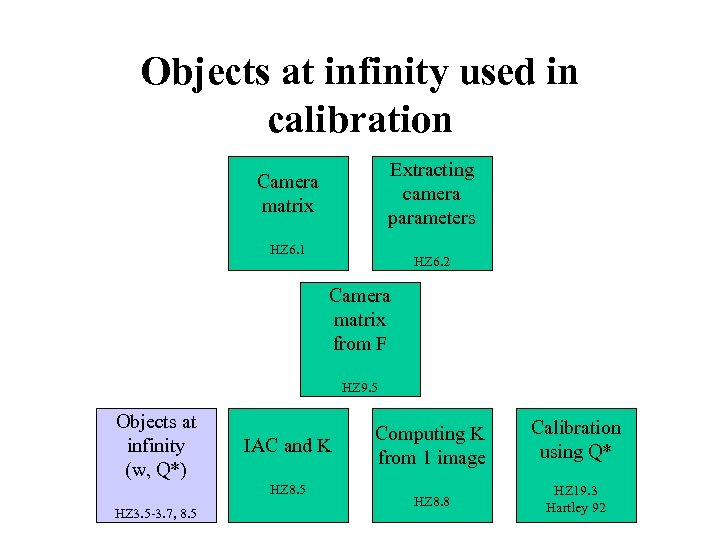Objects at infinity used in calibration Extracting camera parameters Camera matrix HZ 6. 1 HZ 6. 2 Camera matrix from F HZ 9. 5 Objects at infinity (w, Q*) IAC and K HZ 8. 5 HZ 3. 5 -3. 7, 8. 5 Computing K from 1 image Calibration using Q* HZ 8. 8 HZ 19. 3 Hartley 92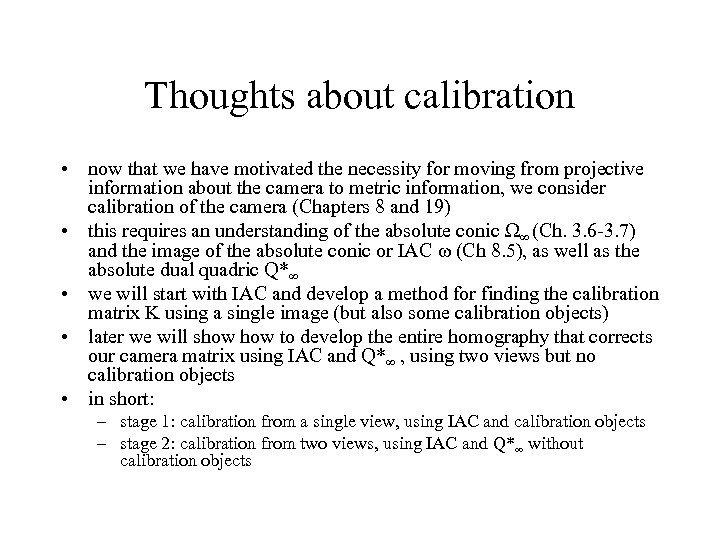Thoughts about calibration • now that we have motivated the necessity for moving from projective information about the camera to metric information, we consider calibration of the camera (Chapters 8 and 19) • this requires an understanding of the absolute conic Ω∞ (Ch. 3. 6 -3. 7) and the image of the absolute conic or IAC ω (Ch 8. 5), as well as the absolute dual quadric Q*∞ • we will start with IAC and develop a method for finding the calibration matrix K using a single image (but also some calibration objects) • later we will show to develop the entire homography that corrects our camera matrix using IAC and Q*∞ , using two views but no calibration objects • in short: – stage 1: calibration from a single view, using IAC and calibration objects – stage 2: calibration from two views, using IAC and Q*∞ without calibration objects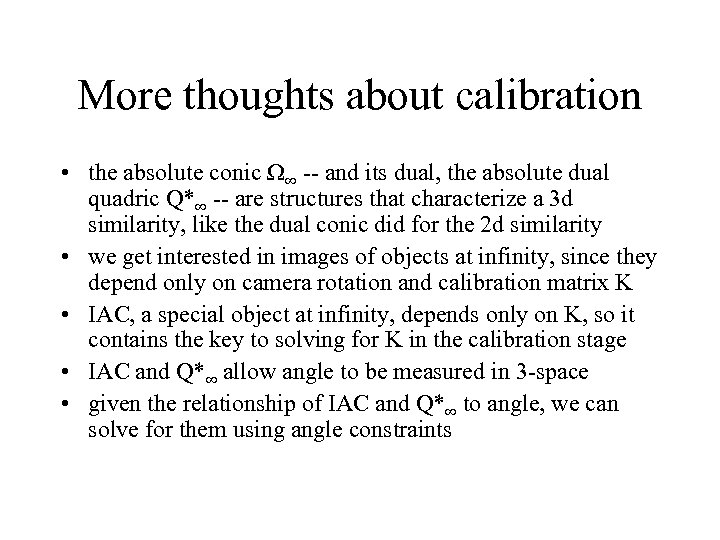More thoughts about calibration • the absolute conic Ω∞ -- and its dual, the absolute dual quadric Q*∞ -- are structures that characterize a 3 d similarity, like the dual conic did for the 2 d similarity • we get interested in images of objects at infinity, since they depend only on camera rotation and calibration matrix K • IAC, a special object at infinity, depends only on K, so it contains the key to solving for K in the calibration stage • IAC and Q*∞ allow angle to be measured in 3 -space • given the relationship of IAC and Q*∞ to angle, we can solve for them using angle constraints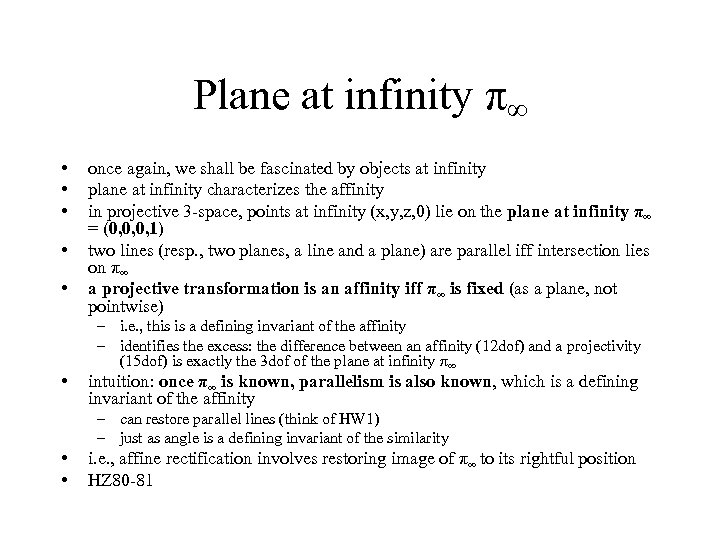Plane at infinity π∞ • • • once again, we shall be fascinated by objects at infinity plane at infinity characterizes the affinity in projective 3 -space, points at infinity (x, y, z, 0) lie on the plane at infinity π∞ = (0, 0, 0, 1) two lines (resp. , two planes, a line and a plane) are parallel iff intersection lies on π∞ a projective transformation is an affinity iff π∞ is fixed (as a plane, not pointwise) – i. e. , this is a defining invariant of the affinity – identifies the excess: the difference between an affinity (12 dof) and a projectivity (15 dof) is exactly the 3 dof of the plane at infinity π∞ intuition: once π∞ is known, parallelism is also known, which is a defining invariant of the affinity – can restore parallel lines (think of HW 1) – just as angle is a defining invariant of the similarity • • i. e. , affine rectification involves restoring image of π∞ to its rightful position HZ 80 -81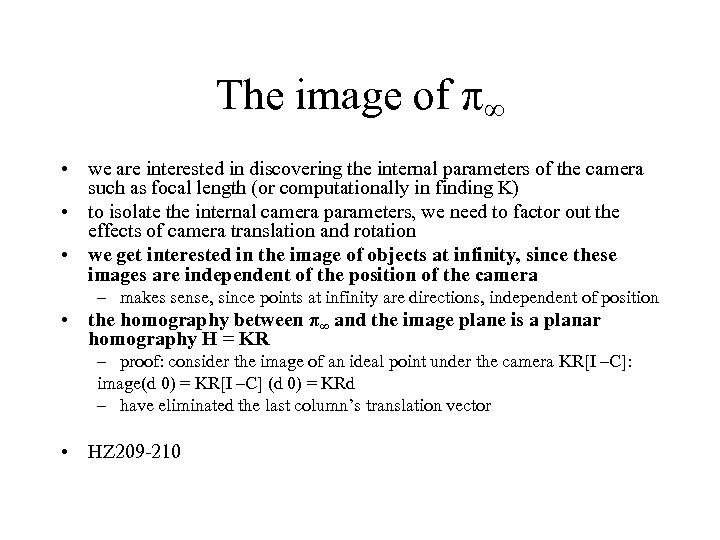The image of π∞ • we are interested in discovering the internal parameters of the camera such as focal length (or computationally in finding K) • to isolate the internal camera parameters, we need to factor out the effects of camera translation and rotation • we get interested in the image of objects at infinity, since these images are independent of the position of the camera – makes sense, since points at infinity are directions, independent of position • the homography between π∞ and the image plane is a planar homography H = KR – proof: consider the image of an ideal point under the camera KR[I –C]: image(d 0) = KR[I –C] (d 0) = KRd – have eliminated the last column’s translation vector • HZ 209 -210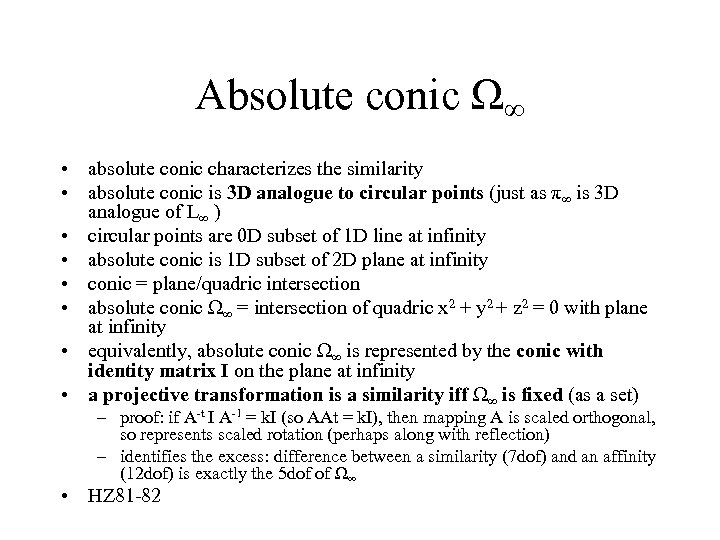Absolute conic Ω∞ • absolute conic characterizes the similarity • absolute conic is 3 D analogue to circular points (just as π∞ is 3 D analogue of L∞ ) • circular points are 0 D subset of 1 D line at infinity • absolute conic is 1 D subset of 2 D plane at infinity • conic = plane/quadric intersection • absolute conic Ω∞ = intersection of quadric x 2 + y 2 + z 2 = 0 with plane at infinity • equivalently, absolute conic Ω∞ is represented by the conic with identity matrix I on the plane at infinity • a projective transformation is a similarity iff Ω∞ is fixed (as a set) – proof: if A-t I A-1 = k. I (so AAt = k. I), then mapping A is scaled orthogonal, so represents scaled rotation (perhaps along with reflection) – identifies the excess: difference between a similarity (7 dof) and an affinity (12 dof) is exactly the 5 dof of Ω∞ • HZ 81 -82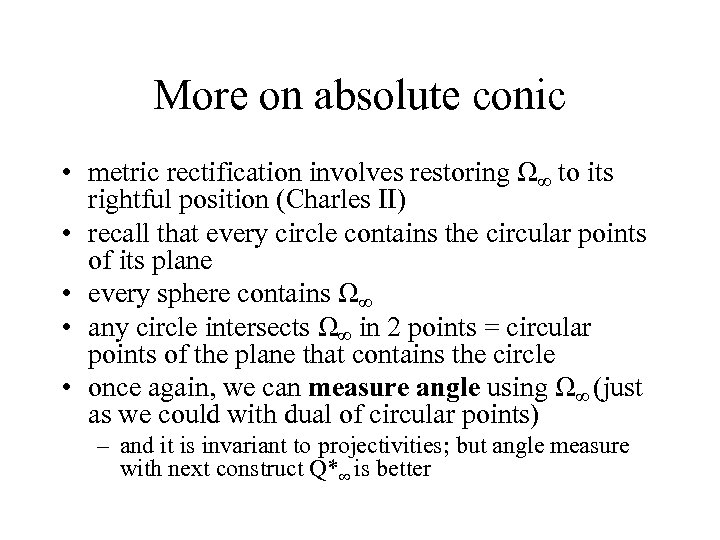More on absolute conic • metric rectification involves restoring Ω∞ to its rightful position (Charles II) • recall that every circle contains the circular points of its plane • every sphere contains Ω∞ • any circle intersects Ω∞ in 2 points = circular points of the plane that contains the circle • once again, we can measure angle using Ω∞ (just as we could with dual of circular points) – and it is invariant to projectivities; but angle measure with next construct Q*∞ is better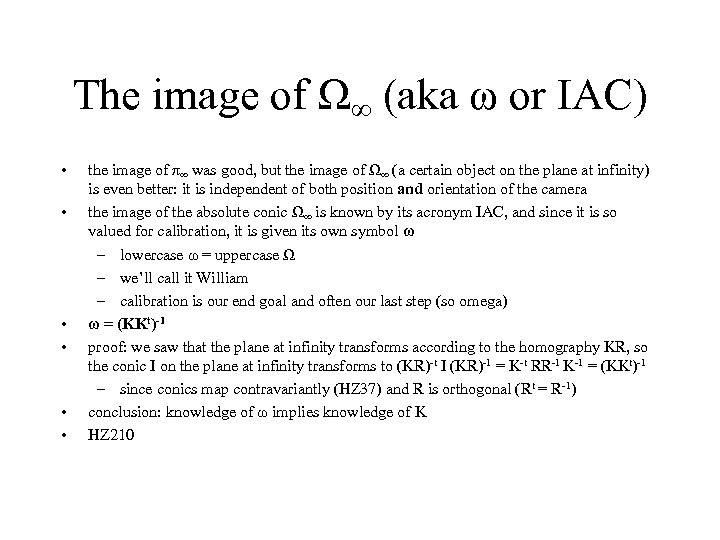The image of Ω∞ (aka ω or IAC) • • • the image of π∞ was good, but the image of Ω∞ (a certain object on the plane at infinity) is even better: it is independent of both position and orientation of the camera the image of the absolute conic Ω∞ is known by its acronym IAC, and since it is so valued for calibration, it is given its own symbol ω – lowercase ω = uppercase Ω – we’ll call it William – calibration is our end goal and often our last step (so omega) ω = (KKt)-1 proof: we saw that the plane at infinity transforms according to the homography KR, so the conic I on the plane at infinity transforms to (KR)-t I (KR)-1 = K-t RR-1 K-1 = (KKt)-1 – since conics map contravariantly (HZ 37) and R is orthogonal (Rt = R-1) conclusion: knowledge of ω implies knowledge of K HZ 210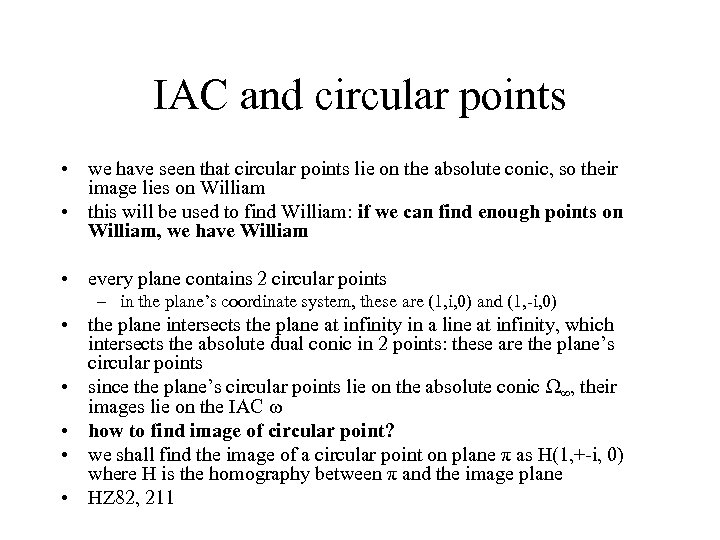IAC and circular points • we have seen that circular points lie on the absolute conic, so their image lies on William • this will be used to find William: if we can find enough points on William, we have William • every plane contains 2 circular points – in the plane’s coordinate system, these are (1, i, 0) and (1, -i, 0) • the plane intersects the plane at infinity in a line at infinity, which intersects the absolute dual conic in 2 points: these are the plane’s circular points • since the plane’s circular points lie on the absolute conic Ω∞, their images lie on the IAC ω • how to find image of circular point? • we shall find the image of a circular point on plane π as H(1, +-i, 0) where H is the homography between π and the image plane • HZ 82, 211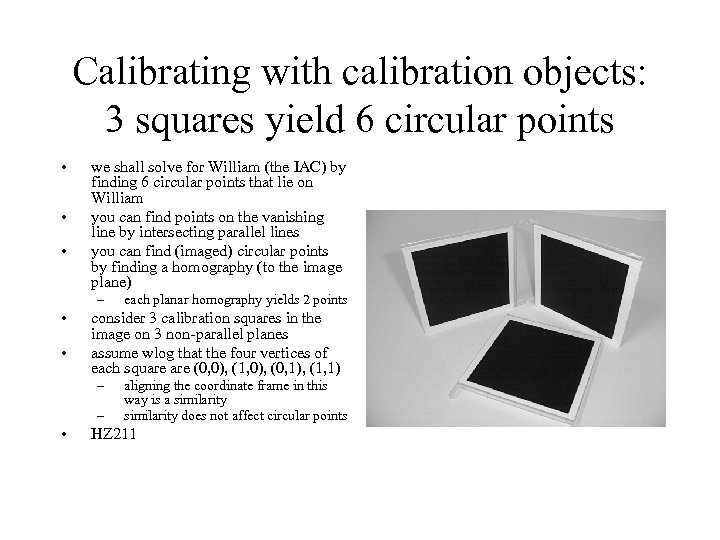Calibrating with calibration objects: 3 squares yield 6 circular points • • • we shall solve for William (the IAC) by finding 6 circular points that lie on William you can find points on the vanishing line by intersecting parallel lines you can find (imaged) circular points by finding a homography (to the image plane) – • • consider 3 calibration squares in the image on 3 non-parallel planes assume wlog that the four vertices of each square (0, 0), (1, 0), (0, 1), (1, 1) – – • each planar homography yields 2 points aligning the coordinate frame in this way is a similarity does not affect circular points HZ 211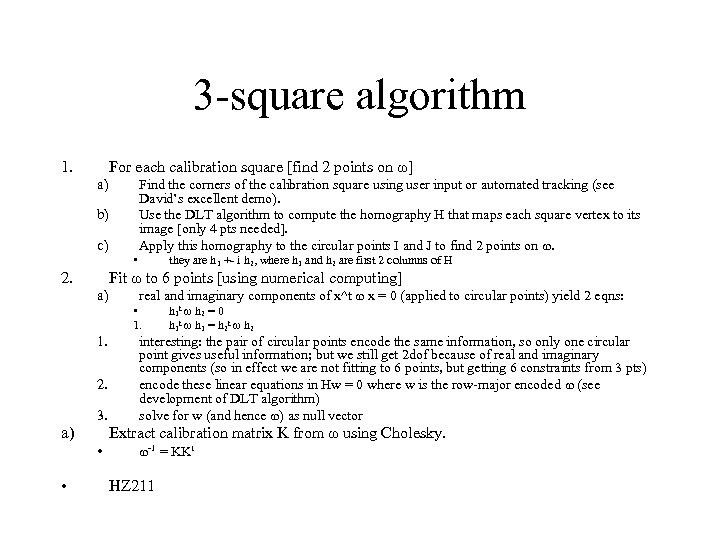3 -square algorithm 1. For each calibration square [find 2 points on ω] a) Find the corners of the calibration square using user input or automated tracking (see David’s excellent demo). Use the DLT algorithm to compute the homography H that maps each square vertex to its image [only 4 pts needed]. Apply this homography to the circular points I and J to find 2 points on ω. b) c) • 2. they are h 1 +- i h 2, where h 1 and h 2 are first 2 columns of H Fit ω to 6 points [using numerical computing] a) real and imaginary components of x^t ω x = 0 (applied to circular points) yield 2 eqns: • 1. 2. 3. a) interesting: the pair of circular points encode the same information, so only one circular point gives useful information; but we still get 2 dof because of real and imaginary components (so in effect we are not fitting to 6 points, but getting 6 constraints from 3 pts) encode these linear equations in Hw = 0 where w is the row-major encoded ω (see development of DLT algorithm) solve for w (and hence ω) as null vector Extract calibration matrix K from ω using Cholesky. • • h 1 t ω h 2 = 0 h 1 t ω h 1 = h 2 t ω h 2 ω-1 = KKt HZ 211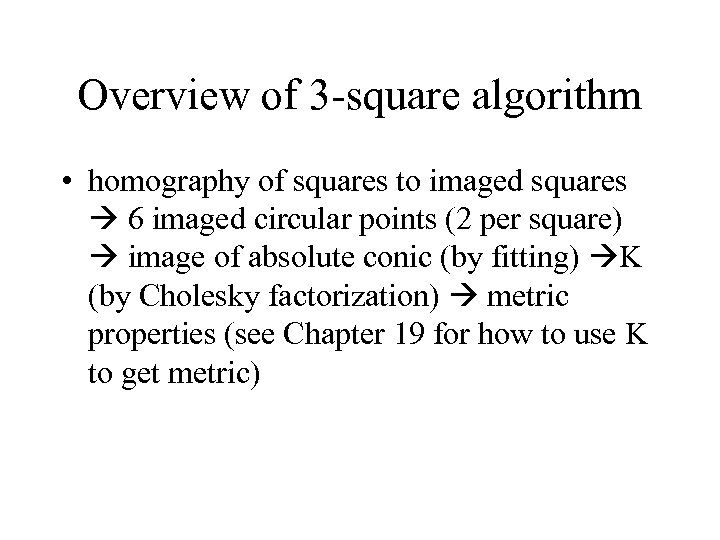Overview of 3 -square algorithm • homography of squares to imaged squares 6 imaged circular points (2 per square) image of absolute conic (by fitting) K (by Cholesky factorization) metric properties (see Chapter 19 for how to use K to get metric)